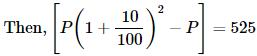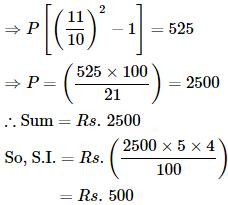Courses

# Test: Compound Interest- 2

## 10 Questions MCQ Test General Aptitude for GATE 2020 | Test: Compound Interest- 2

Description
This mock test of Test: Compound Interest- 2 for Quant helps you for every Quant entrance exam. This contains 10 Multiple Choice Questions for Quant Test: Compound Interest- 2 (mcq) to study with solutions a complete question bank. The solved questions answers in this Test: Compound Interest- 2 quiz give you a good mix of easy questions and tough questions. Quant students definitely take this Test: Compound Interest- 2 exercise for a better result in the exam. You can find other Test: Compound Interest- 2 extra questions, long questions & short questions for Quant on EduRev as well by searching above.
QUESTION: 1

### The Simple interest on a certain sum for 2 years at 20% per annum is Rs. 80. The corresponding compound interest is

Solution:

P = (100×80)/(20×2) = 200
20%(200)= 40
20%(40) = 8
Required compound interest = 2(40)+ 8
= 88

QUESTION: 2

### The compound interest on a certain sum for 2 years at 10% per annum is Rs. 525. The simple interest on the same sum for double the time at half the rate percent per annum is:

Solution:

Let the sum be Rs. PQUESTION: 3

### The effective annual rate of interest corresponding to a nominal rate of 6% per annum payable half-yearly is:

Solution:
QUESTION: 4

The compound interest on Rs. 20,480 at 6 1/4 % per annum for 2 years 73 days, is:

Solution:
QUESTION: 5

A sum put out at 4% compound interest payable half-yearly amounts to Rs. 13265.10 in 1 1/2 years. The sum is:

Solution:
QUESTION: 6

Arun invested an amount of Rs. 20000 in a fixed deposit scheme for 2 years at compound interest rate 4 p.c.p.a. How much amount will Arun get on maturity of the fixed deposit?

Solution:
QUESTION: 7

Simple interest on a certain sum of money for 4 years at 5% per annum is half the compound interest on Rs. 3000 for 2 years at 10% per annum. The sum placed on simple interest is:

Solution:

C.I = P[(1 + r/100)n - 1]
C.I = 3000[(1 + 0.10)2 - 1]
= 3000(1.21 - 1)
⇒ 3000 * 0.21
⇒ 630
S.I = 630/2
⇒ 315
As we know that, P = (SI * 100)/(R * T)
⇒ (315 * 100)/(4 * 5)
⇒ 1575

QUESTION: 8

A sum amounts to Rs. 882 in 2 years at 5% compound interest. The sum is

Solution:

Compound interest = Amount -Principal
Amount = P(1 + R/100)N
882 = P(1+5/100)2
=  882 × 20/21 × 20/21
P = 800

QUESTION: 9

The least number of complete years in which a sum of money put out at 20% compound interest will be more than doubled is

Solution:

P [1 + (r/100)]n  >  2P
⇒ P [1 + (20/100)]n  >  2P
[1 + (2/10) ]n  > 2
[12 / 10]n  > 2
[6/5]n  > 2
{6/5 * 6/5 * 6/5 * 6/5}  >  2
∴ n = 4

QUESTION: 10

The difference between simple interest and compound on Rs. 2400 for one year at 10% per annum reckoned half-yearly is:

Solution: